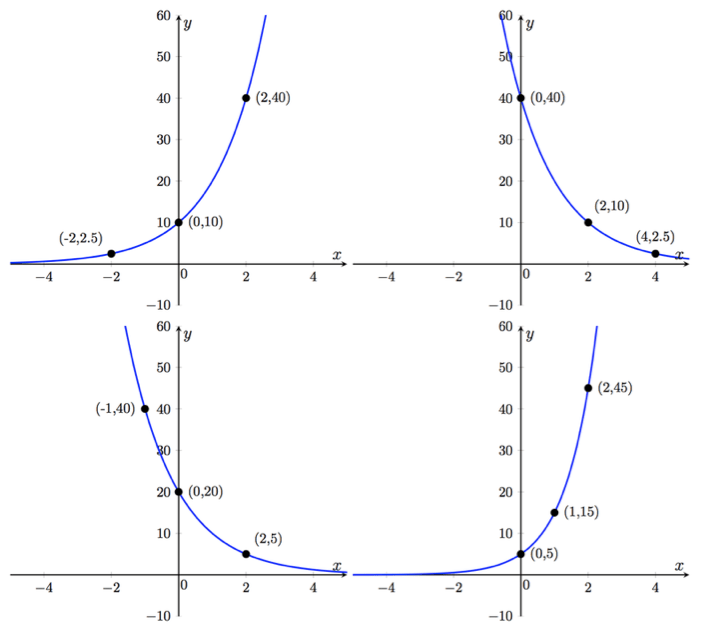# FNGR 1 | Lesson 2 | Practice (Comparing Exponential Growth and Decay)# Comparing Exponential Growth and DecayIdentify the four graphs as either exponential growth or exponential decay.

What are the initial values for each of the graphs?

How might you determine the ratio of which each of the graphs is changing?

How might you determine the algebraic representation of each of the graphs?

Go to Illustrative Mathematics to see image and solutions.